×#### Thank you for registering.

One of our academic counsellors will contact you within 1 working day.

Click to Chat

1800-1023-196

+91-120-4616500

CART 0

• 0

MY CART (5)

Use Coupon: CART20 and get 20% off on all online Study Material

ITEM
DETAILS
MRP
DISCOUNT
FINAL PRICE
Total Price: Rs.

There are no items in this cart.
Continue Shopping• Complete JEE Main/Advanced Course and Test Series
• OFFERED PRICE: Rs. 15,900
• View Details

```Chapter 20: Linear Equation in One Variable Exercise – 20.2

Question: 1

Find the area, in square metres, of the trapezium whose bases and altitudes are as under:

(i) bases = 12 dm and 20 dm, altitude = 10 dm

(ii) bases = 28 cm and 3 dm, altitude = 25 cm

(iii) bases = 8 m and 60 dm, altitude = 40 dm

(iv) bases = 150 cm and 30 dm, altitude = 9 dm.

Solution:

(i) Given:

Bases: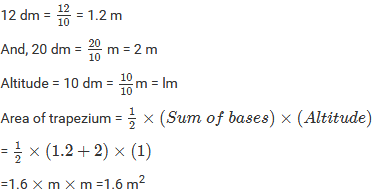(ii)

Given:

Bases: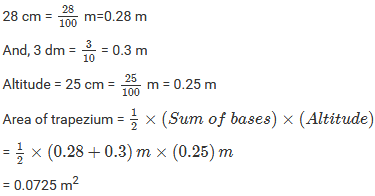(iii)

Given:

Bases: 8m(iv)

Given: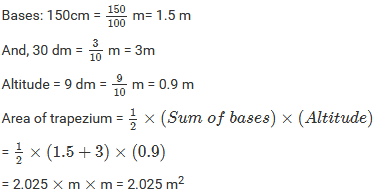Question: 2

Find the area of trapezium with base 15 cm and height 8 cm, if the side parallel to the given base is 9 cm long.

Solution:

Given: Lengths of the parallel sides are 15 cm and 9 cm.

Height = 8 cm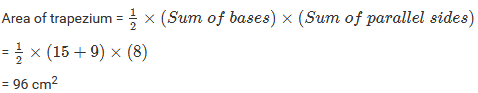Question: 3

Find the area of a trapezium whose parallel sides are of length 16 dm and 22 dm and whose height is 12 dm.

Solution:

Given:

Lengths of the parallel sides are 16 dm and 22 dm.

And, height between the parallel sides is 12 dm.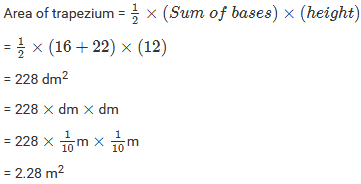Question: 4

Find the height of a trapezium, the sum of the lengths of whose bases (parallel sides) is 60 cm and whose area is 600 cm2.

Solution:

Given:

Sum of the parallel sides of a trapezium = 60 cm

Area of the trapezium = 600 cm2Question: 5

Find the altitude of a trapezium whose area is 65 cm2 and whose bases are 13 cm and 26 cm.

Solution:

Given:

Area of the trapezium = 65 cm2

The lengths of the opposite parallel sides are 13 cm and 26 cm.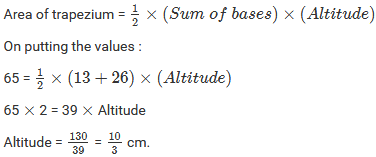Question: 6

Find the sum of the length of the bases of a trapezium whose area is 4.2 m2 and whose height is 280 cm.

Solution:

Given:

Area of the trapezium = 4.2 m2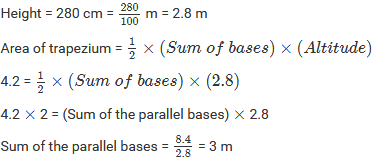Question: 7

Find the area of a trapezium whose parallel sides of lengths 10 cm and 15 cm are at a distance of 6 cm from each other. Calculate this area as:

(i) the sum of the areas of two triangles and one rectangle.

(ii) the difference of the area of a rectangle and the sum of the areas of two triangles.

Solution:

Given: Length of the parallel sides of a trapezium are 10 cm and 15 cm.

The distance between them is 6 cm.

Let us extend the smaller side and then draw perpendiculars from the ends of both sides.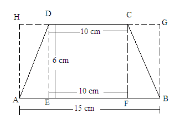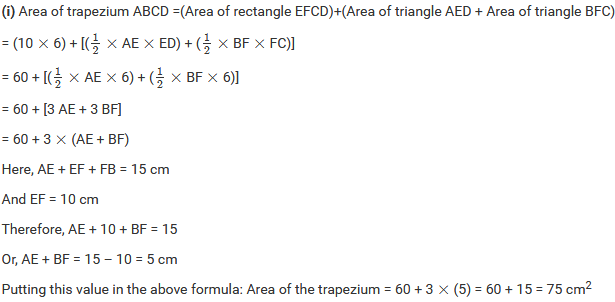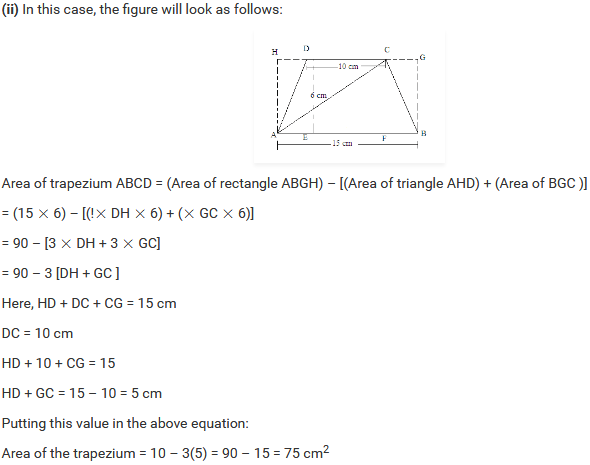Question: 8

The area of a trapezium is 960 cm2. If the parallel sides are 34 cm and 46 cm, find the distance between them.

Solution:

Given:

Area of the trapezium = 960 cm2

And the length of the parallel sides are 34 cm and 46 cm.Question: 9

Find the area of Figure below as the sum of the areas of two trapeziums and a rectangle.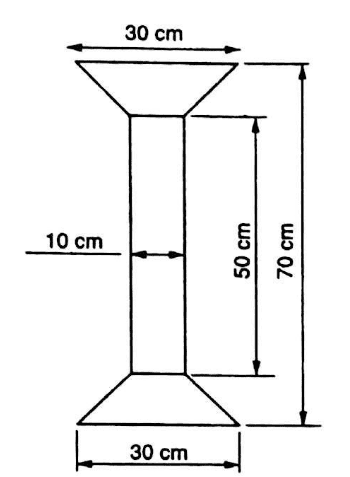Solution:

In the given figure, we have a rectangle of length 50 cm and width 10 cm and two similar

trapeziums with parallel sides as 30 cm and 10 cm at both ends.

Suppose x is the perpendicular distance between the parallel sides in both the trapeziums.

We have:

Total length of the given figure = Length of the rectangle + 2 × Perpendicular distance between the parallel sides in both the trapeziums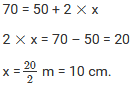Now, area of the complete figure = (area of the rectangle with sides 50 cm and 10 cm) + 2 × (area of the trapezium with parallel sides 30 cm and 10 cm, and height 10 cm)

= (50 × 10) + 2 × [ 1/2 × (30 + 10) × (10)] = 500 + 2 ×  = 900 cm2.

Question: 10

Top surface of a table is trapezium in shape. Find its area if its parallel sides are 1 m and 1.2 m and perpendicular distance between them is 0.8 m.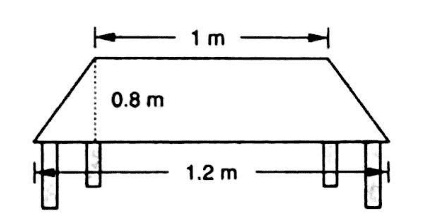Solution:

The given figure is:Question: 11

The cross-section of a canal is a trapezium in shape. If the canal is 10 m wide at the top 6 m wide at the bottom and the area of cross-section is 72 m2 determine its depth.

Solution:

Let the depth of canal bke d.

Given:

Lengths of the parallel sides of the trapezium shape canal are 10 m and 6 m.

And, the area of the cross-section of the canal is 72 m2.Question: 12

The area of a trapezium is 91 c m2 and its height is 7 cm. If one of the parallel sides is longer than the other by 8 cm, find the two parallel sides.

Solution:

Given:

Area of the trapezium = 91 cm2

Height = 7 cm

Let the length of the smaller side be x.

Then, the length of the longer side will be 8 more than the smaller side, i.e. 8 + x.Therefore, Length of the shorter side of the trapezium = 9 cm

And, length of the longer side = 8 + x = 8 + 9 = 17 cm.

Question: 13

The area of a trapezium is 384 c m2. Its parallel sides are in the ratio 3: 5 and the perpendicular distance between them is 12 cm. Find the length of each one of the parallel sides.

Solution:

Given:

Area of the trapezium = 384 cm2

The parallel sides are in the ratio 3:5 and the perpendicular height between them is 12 cm.

Suppose that the sides are in x multiples of each other.

Then, length of the shorter side = 3x

Length of the longer side = 5x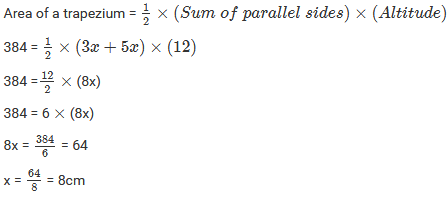Therefore, Length of the shorter side = 3 × x = 3 × 8 = 24 cm

And, length of the longer side = 5 × 5 × 8 = 40 cm.

Question: 14

Mohan wants to buy a trapezium-shaped field. Its side along the river is parallel and twice the side of the road. If the area of this field is 10500 m2 and the perpendicular distance between the two parallel sides is 100 m, find the length of the side along the river.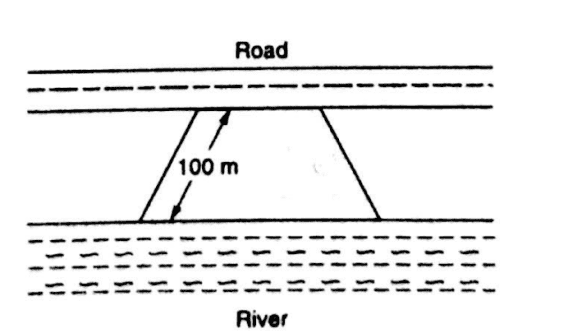Solution:

Given:

Area of the trapezium-shaped field = 10500 m2

It is also given that the length of the side along the river is double the length of the side ;

Let us suppose the length of the side along the road to be x.

Then, the length of the side along the river = 2 × x = 2x

And, the perpendicular distance between these parallel sides = 100 m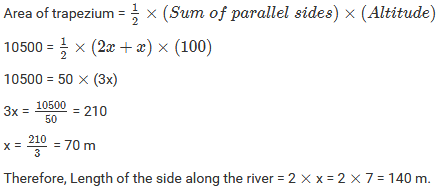Question: 15

The area of a trapezium is 1586 c m2 and the distance between the parallel sides is 26 cm. If one of the parallel sides is 38 cm, find the other.

Solution:

Given:

Area of the trapezium = 1586 cm2

Distance between the parallel sides = 26 cm

And, length of one parallel side = 38 cm

Let us suppose the length of the other side to be x cm.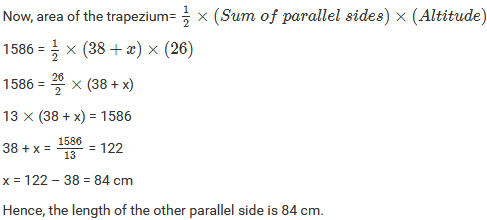Question: 16

The parallel sides of a trapezium are 25 cm and 13 cm; its nonparallel sides are equal, each being 10 cm, find the area of the trapezium.

Solution:

Given: The parallel sides of a trapezium are 25 cm and 13 cm.

Its nonparallel sides are equal in length and each is equal to 10 cm.

A rough sketch for the given trapezium is given below: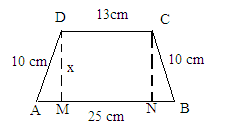In above figure, we observe that both the right angle triangles AMD and BNC are congruent triangles.

AD = BC = 10 cm

D = CN = x cm

∠DMA = ∠CNB = 90∘

Hence, the third side of both the triangles will also be equal.

Therefore, AM = BN

Also, MN = 13

Since AB = AM + MN + NB

Therefore, 25 = AM + 13 + BN

AM + BN = 25 – 13 = 12 cm

Or, BN + BN = 12 cm (Because AM=I3N)

2 BN = 12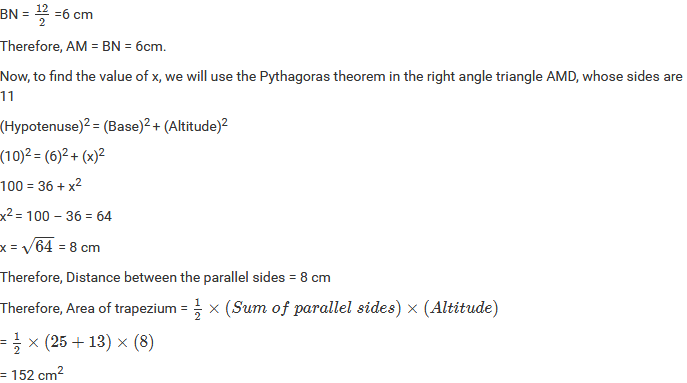Question: 17

Find the area of a trapezium whose parallel sides are 25 cm, 13 cm and the other sides are 15 cm each.

Solution:

Given: Parallel sides of a trapezium are 25 cm and 13 cm.

Its nonparallel sides are equal in length and each is equal to 15 cm.

A rough sketch of the trapezium is given below: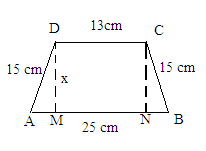In above figure, we observe that both the right angle triangles AMD and BNC are similar

This is because both have two common sides as 15 cm and the altitude as x and a right angle.

Hence, the remaining side of both the triangles will be equal.

Therefore, AM = BN

Also MN = 13

Now, since AB = AM + MN + NB:

Therefore, 25 = AM + 13 + BN

AM + BN = 25 – 13 = 12 cm

Or, BN + BN = 12 cm (Because AM = BN)

2 BN = 12

BN = 12/2 = 6 cm

AM = BN=6 cm

Now, to find the value of x, we will use the Pythagorean theorem in the right angle triangle AMD whose sides are 15, 6 and x.

(Hypotenuse)2 = (Base)2– (Altitude)2

(15)2 = (6)2 + (x)2

225 = 36 + (x)2

(x)2 = 225 – 36 = 189Question: 18

If the area of a trapezium is 28 cm2 and one of its parallel sides is 6 cm, find the other parallel side if its altitude is 4 cm.

Solution:

Given: Area of the trapezium = 28 cm2

Length of one of its parallel sides = 6 cm

Altitude = 4 cm

Let the other side be x cm.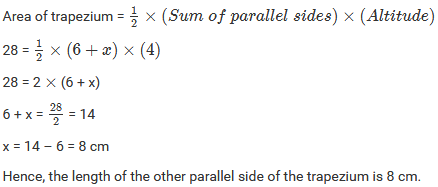Question: 19

In Figure below, a parallelogram is drawn in a trapezium, the area of the parallelogram is 80 cm2, find the area of the trapezium.Solution:

The given figure is:From above figure, it is clear that the length of the parallel sides of the trapezium are 22 cm and 10 cm.

Also, it is given that the area of the parallelogram is 80 cm2 and its base is 10 cm.

We know:

Area of parallelogram = Base × Height

80 = 10 × Height

Height = 80/10 = 8 cm

So, now we have the distance between the parallel sides of trapezium, which is equal to 8 cm.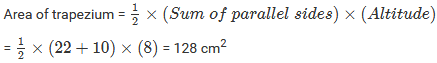Question: 20

Find the area of the field shown in Figure below by dividing it into a square, a rectangle and a trapezium.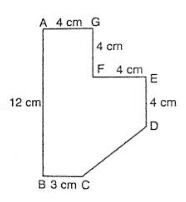Solution:

The given figure can be divided into a square, a parallelogram and a trapezium as shown in figure.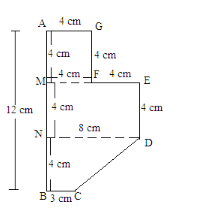From the above figure: Area of the figure(Area of square AGFM with sides 4 cm) + (Area of rectangle MEDN with length 8 cm and width 4 cm) + (Area of trapezium NDCB with parallel sides 8 cm and 3 cm and perpendicular height 4 cm)

= (4 × 4) + ( 8 × 4) + [ 1/2 × (8 + 3) × (4)] = 16 + 32 + 22 = 70 cm2
```### Course Features

• 728 Video Lectures
• Revision Notes
• Previous Year Papers
• Mind Map
• Study Planner
• NCERT Solutions
• Discussion Forum
• Test paper with Video Solution